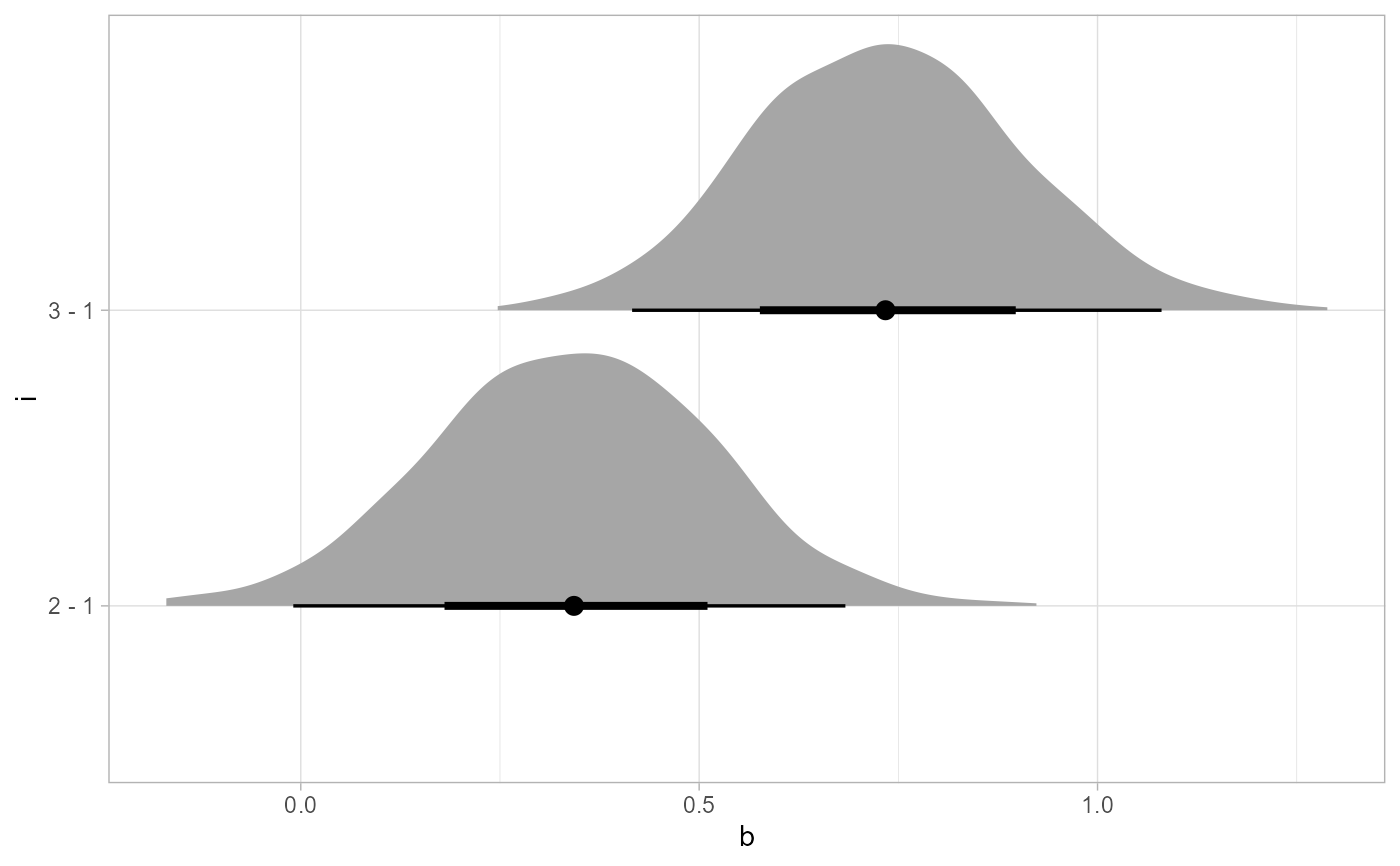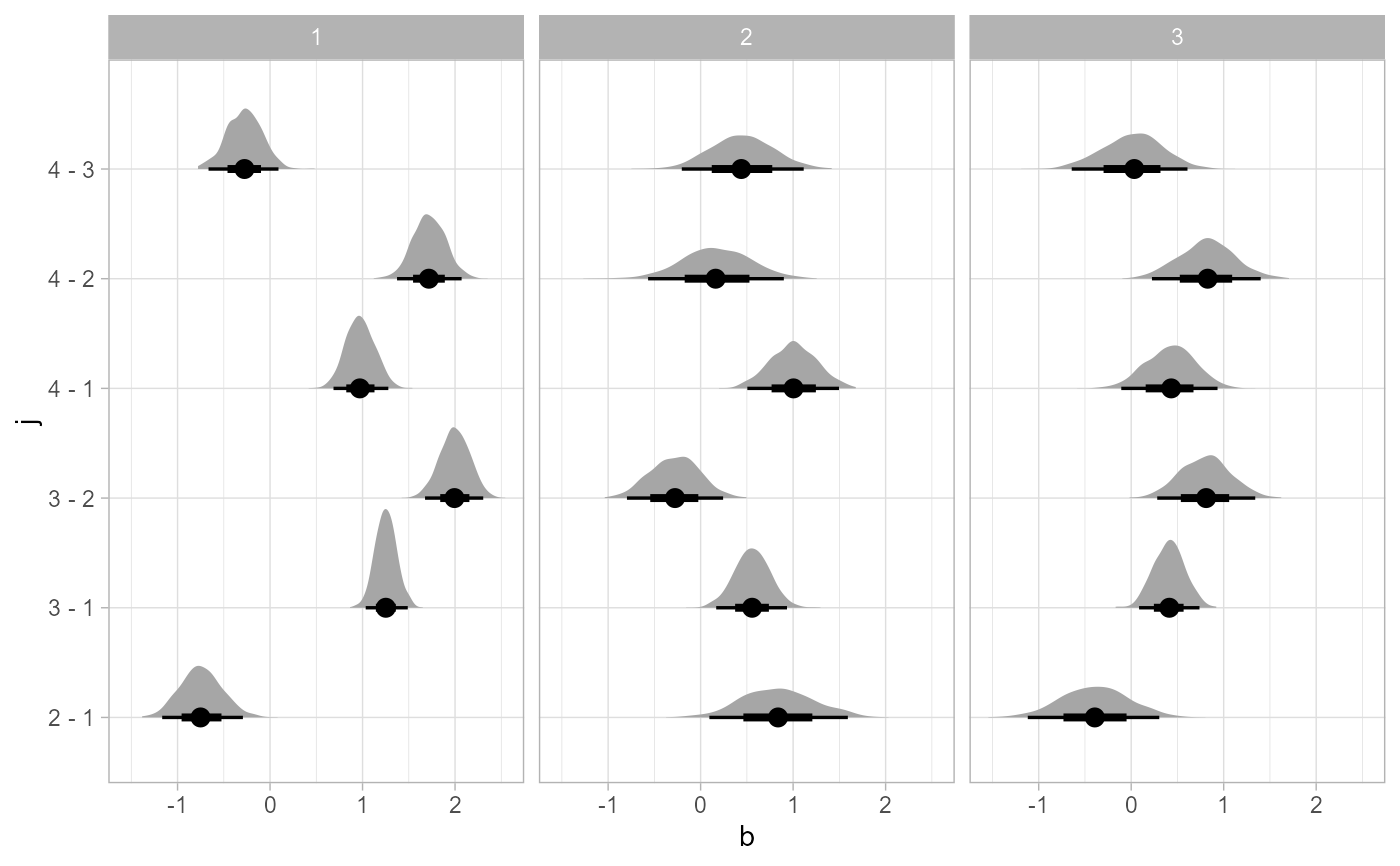Given posterior draws from a Bayesian model in long format (e.g. as returned by spread_draws()), compare the value of a variable in those draws across different paired combinations of levels of a factor.

compare_levels(
data,
variable,
by,
fun = -,
comparison = "default",
draw_indices = c(".chain", ".iteration", ".draw"),
ignore_groups = ".row"
)

## Arguments

data

Long-format data.frame of draws such as returned by spread_draws() or gather_draws(). If data is a grouped data frame, comparisons will be made within groups (if one of the groups in the data frame is the by column, that specific group will be ignored, as it is not possible to make comparisons both within some variable and across it simultaneously).

variable

Bare (unquoted) name of a column in data representing the variable to compare across levels. Can be a numeric variable (as in long-data-frame-of-draws format) or a posterior::rvar.

by

Bare (unquoted) name of a column in data that is a factor or ordered. The value of variable will be compared across pairs of levels of this factor.

fun

Binary function to use for comparison. For each pair of levels of by we are comparing (as determined by comparison), compute the result of this function.

comparison

One of (a) the comparison types ordered, control, pairwise, or default (may also be given as strings, e.g. "ordered"), see Details; (b) a user-specified function that takes a factor and returns a list of pairs of names of levels to compare (as strings) and/or unevaluated expressions containing representing the comparisons to make; or (c) a list of pairs of names of levels to compare (as strings) and/or unevaluated expressions representing the comparisons to make, e.g.: list(c("a", "b"), c("b", "c")) or exprs(a - b, b - c), both of which would compare level "a" against "b" and level "b" against "c". Note that the unevaluated expression syntax ignores the fun argument, can include any other functions desired (e.g. variable transformations), and can even include more than two levels or other columns in data. Types (b) and (c) may use named lists, in which case the provided names are used in the output variable column instead converting the unevaluated expression to a string. You can also use emmeans_comparison() to generate a comparison function based on contrast methods from the emmeans package.

draw_indices

Character vector of column names in data that should be treated as indices when making the comparison (i.e. values of variable within each level of by will be compared at each unique combination of levels of draw_indices). Columns in draw_indices not found in data are ignored. The default is c(".chain",".iteration",".draw"), which are the same names used for chain/iteration/draw indices returned by spread_draws() or gather_draws(); thus if you are using compare_levels with spread_draws() or gather_draws() you generally should not need to change this value.

ignore_groups

character vector of names of groups to ignore by default in the input grouping. This is primarily provided to make it easier to pipe output of add_epred_draws() into this function, as that function provides a ".row" output column that is grouped, but which is virtually never desired to group by when using compare_levels.

## Value

A data.frame with the same columns as data, except that the by column contains a symbolic representation of the comparison of pairs of levels of by in data, and variable contains the result of that comparison.

## Details

This function simplifies conducting comparisons across levels of some variable in a tidy data frame of draws. It applies fun to all values of variable for each pair of levels of by as selected by comparison. By default, all pairwise comparisons are generated if by is an unordered factor and ordered comparisons are made if by is ordered.

The included comparison types are:

• ordered: compare each level i with level i - 1; e.g. fun(i, i - 1)

• pairwise: compare each level of by with every other level.

• control: compare each level of by with the first level of by. If you wish to compare with a different level, you can first apply relevel() to by to set the control (reference) level.

• default: use ordered if is.ordered(by) and pairwise otherwise.

emmeans_comparison() to use emmeans-style contrast methods with compare_levels().

Matthew Kay

## Examples


library(dplyr)
library(ggplot2)

data(RankCorr, package = "ggdist")

# Let's do all pairwise comparisons of b[i,1]:
RankCorr %>%
filter(j == 1) %>%
compare_levels(b, by = i) %>%
median_qi()
#> # A tibble: 3 x 8
#>   i         j     b   .lower .upper .width .point .interval
#>   <chr> <int> <dbl>    <dbl>  <dbl>  <dbl> <chr>  <chr>
#> 1 2 - 1     1 0.343 -0.00937  0.684   0.95 median qi
#> 2 3 - 1     1 0.734  0.416    1.08    0.95 median qi
#> 3 3 - 2     1 0.402 -0.0133   0.791   0.95 median qi

# Or let's plot all comparisons against the first level (control):
RankCorr %>%
filter(j == 1) %>%
compare_levels(b, by = i, comparison = control) %>%
ggplot(aes(x = b, y = i)) +
stat_halfeye()# Or let's plot comparisons of all levels of j within
# all levels of i
RankCorr %>%